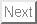Up: NAMD Tutorial Previous: NAMD Standard Output

# Water Sphere tcl Script

```### Script to immerse ubiquitin in a sphere of water just large enough
### to cover it

set molname ubq

mol new \${molname}.psf

### Determine the center of mass of the molecule and store the coordinates
set cen [measure center [atomselect top all] weight mass]
set x1 [lindex \$cen 0]
set y1 [lindex \$cen 1]
set z1 [lindex \$cen 2]
set max 0

### Determine the distance of the farthest atom from the center of mass
foreach atom [[atomselect top all] get index] {
set pos [lindex [[atomselect top "index \$atom"] get {x y z}] 0]
set x2 [lindex \$pos 0]
set y2 [lindex \$pos 1]
set z2 [lindex \$pos 2]
set dist [expr pow((\$x2-\$x1)*(\$x2-\$x1) + (\$y2-\$y1)*(\$y2-\$y1) +
(\$z2-\$z1)*(\$z2-\$z1),0.5)]
if {\$dist > \$max} {set max \$dist}
}

mol delete top

### Solvate the molecule in a water box with enough padding (15 A).
### One could alternatively align the molecule such that the vector
### from the center of mass to the farthest atom is aligned with an axis,
### and then use no padding
package require solvate
solvate \${molname}.psf \${molname}.pdb -t 15 -o del_water

resetpsf
package require psfgen
mol new del_water.psf
coordpdb del_water.pdb

### Determine which water molecules need to be deleted and use a for loop
### to delete them
set wat [atomselect top "same residue as {water and ((x-\$x1)*(x-\$x1) +
(y-\$y1)*(y-\$y1) + (z-\$z1)*(z-\$z1))<(\$max*\$max)}"]
set del [atomselect top "water and not same residue as {water and
((x-\$x1)*(x-\$x1) + (y-\$y1)*(y-\$y1) + (z-\$z1)*(z-\$z1))<(\$max*\$max)}"]
set seg [\$del get segid]
set res [\$del get resid]
set name [\$del get name]
for {set i 0} {\$i < [llength \$seg]} {incr i} {
delatom [lindex \$seg \$i] [lindex \$res \$i] [lindex \$name \$i]
}
writepsf \${molname}_ws.psf
writepdb \${molname}_ws.pdb

mol delete top

mol new \${molname}_ws.psf